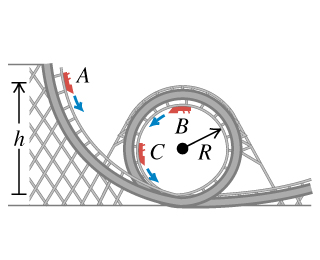# Problem: A car in an amusement park ride rolls without friction around a track . The car starts from rest at point A at a height h above the bottom of the loop. Treat the car as a particle.What is the minimum value of h (in terms of R) such that the car moves around the loop without falling off at the top (point B)?If the car starts at height h= 4.10 R and the radius is exttip{R_{ m 1}}{R_1} = 15.0 m , compute the speed of the passengers when the car is at point C, which is at the end of a horizontal diameter.Compute the radial acceleration of the passengers when the car is at point C, which is at the end of a horizontal diameter.Compute the tangential acceleration of the passengers when the car is at point C, which is at the end of a horizontal diameter.

###### FREE Expert Solution
92% (443 ratings)
###### Problem Details

A car in an amusement park ride rolls without friction around a track. The car starts from rest at point A at a height h above the bottom of the loop. Treat the car as a particle.

What is the minimum value of h (in terms of R) such that the car moves around the loop without falling off at the top (point B)?

If the car starts at height h= 4.10 R and the radius is = 15.0 m , compute the speed of the passengers when the car is at point C, which is at the end of a horizontal diameter.

Compute the radial acceleration of the passengers when the car is at point C, which is at the end of a horizontal diameter.

Compute the tangential acceleration of the passengers when the car is at point C, which is at the end of a horizontal diameter.

Frequently Asked Questions

What scientific concept do you need to know in order to solve this problem?

Our tutors have indicated that to solve this problem you will need to apply the Motion Along Curved Paths concept. You can view video lessons to learn Motion Along Curved Paths. Or if you need more Motion Along Curved Paths practice, you can also practice Motion Along Curved Paths practice problems.